#### 期刊菜单

The Analysis of Pointing Error Extraction and Its Tolerant Calibration for a Large Radio Telescope
DOI: 10.12677/JAST.2021.94016, PDF, HTML, XML, 下载: 131  浏览: 344  科研立项经费支持

Abstract: As one of the most important characteristics for a radio telescope, the pointing accuracy of the antenna directly affects the observation performance of the radio telescope. The pointing measurements are collected via cross-scan method in the antenna’s hemispherical sky area, and the pointing error is extracted based on the Gaussian model. In this thesis, the error sources of the antenna pointing are studied and then analyzed the adopted correction methods. The coefficient values of the correction model are obtained by least squares method and a fault-tolerant correction method based on residual statistical test is proposed to verify the error observation sequence and eliminate outliers. Based on the theory of statistical hypothesis test, F-test algorithm and t-test algorithm are built to test the fitness of correction model and diagnose the significance of the model components. Several experiments with measured data demonstrate that the modified parameter model for the pointing corrections from 33.46" to 26.39". The hypothesis test can be used to identify the validity of error model and model components.

1. 引言

2. 射电望远镜指向误差测量及提取

2.1. 指向数据采集Figure 1. Diagram of cross scanning method

1) 在天球上的位置精确已知，并在天球上有较均匀的分布和覆盖较大的分布区域；

2) 对被测射电望远镜的频段内，有足够的信噪比，即射电源在所测频段内具有较大流量密度；

3) 对被射电望远镜天线波束宽度而言，射电源角径应相对较小，即可被视为点源目标；

4) 附近没有其它较强的射电源或银河背景辐射。

2.2. 指向误差提取

$f\left(x\right)=a\ast {\text{e}}^{-{\left(\left(x-b\right)/c\right)}^{2}}$ (1)

$f\left(x\right)=a\ast {\text{e}}^{-{\left(\left(x-b\right)/c\right)}^{2}}+d$ (2)

d0}。然后选择坐标为 $\left[{b}_{0}-\text{3}{c}_{0},{b}_{0}+\text{3}{c}_{0}\right]$ 所在的区间数据作为有效采样数据进行数据拟合。

${R}^{2}=1-SSE/SST$ (3)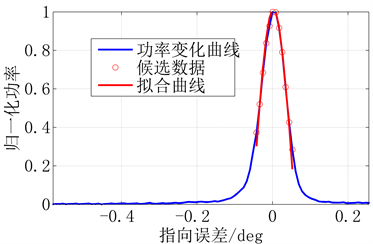(a) 有效提取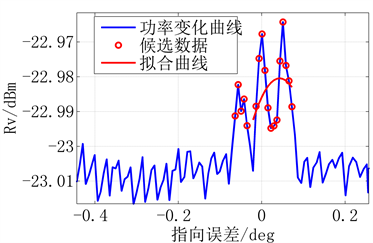(b) 无效提取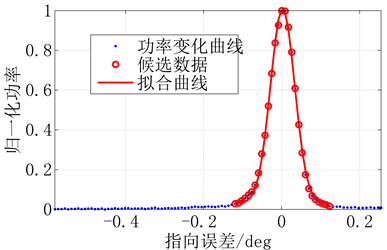(c) R2 = 0.9985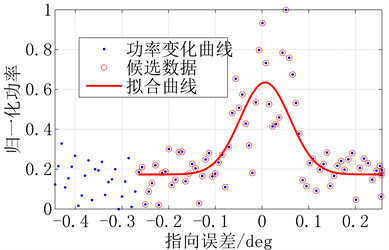(d) R2 = 0.6443

Figure 2. Diagram of pointing error extraction cross: (a) (b) “method 1”; (c) (d) “method 2”

3. 指向误差源分解与建模

3.1. 主要误差源概述

$\begin{array}{l}\Delta A={C}_{1}-{C}_{3}\mathrm{cos}{C}_{4}\mathrm{cos}A\mathrm{tan}E+{C}_{5}\mathrm{tan}E-{C}_{3}\mathrm{sin}{C}_{4}\mathrm{sin}A\mathrm{tan}E-{C}_{6}\mathrm{sec}E+{\epsilon }_{A}\\ \Delta E={C}_{2}+{C}_{3}\mathrm{cos}{C}_{4}\mathrm{sin}A+{C}_{7}\mathrm{cos}E-{C}_{3}\mathrm{sin}{C}_{4}\mathrm{cos}A+{C}_{8}\mathrm{cot}E+{\epsilon }_{E}\end{array}$ (4)

$y=X\beta +\epsilon$ (5)

$y={\left({y}_{1},{y}_{2},\cdots ,{y}_{n}\right)}^{\text{T}}$ 为观测向量；

$X=\left(\begin{array}{ccc}{x}_{11}& \cdots & {x}_{1p}\\ ⋮& \ddots & ⋮\\ {x}_{n1}& \cdots & {x}_{np}\end{array}\right)$$n\ast p$ 阶设计矩阵；

$\beta ={\left({\beta }_{1},{\beta }_{2},\cdots ,{\beta }_{p}\right)}^{\text{T}}$ 为待估计的模型参数向量。

$\stackrel{⌢}{\beta }={\left({X}^{\text{T}}X\right)}^{-1}Xy$ (6)

3.2. 观测值容错修正模型

$e=y-X\stackrel{⌢}{\beta }=y-X{\left({X}^{\text{T}}X\right)}^{-1}Xy\triangleq \left(I-H\right)y$ (7)

${\stackrel{⌢}{\sigma }}_{i}^{2}=\frac{{{e}^{\prime }}_{i}{e}_{i}}{n-p-1}$ (8)

${T}_{i}=\frac{{e}_{i}}{{\stackrel{⌢}{\sigma }}_{i}\sqrt{1-{h}_{ii}}}$ (9)

${T}_{i}>{t}_{\alpha /2}$ (10)

3.3. 误差模型的方程显著性检验

$\left\{\begin{array}{l}{H}_{0}:{\beta }_{1}={\beta }_{2}=\cdots ={\beta }_{p}=0\\ {H}_{1}:{\beta }_{1},{\beta }_{2},\cdots ,{\beta }_{p}\text{ }\text{ }不全为\text{ }\text{ }0\end{array}$ (11)

$F=\frac{SSE/\left(p-1\right)}{SSR/\left(n-p\right)}$ (12)

$F>{F}_{\alpha }\left(p-1,n-p\right)$ (13)

3.4. 误差模型的分量显著性检验

$\left\{\begin{array}{l}{H}_{0}:{\beta }_{j}=0\\ {H}_{1}:{\beta }_{j}\ne 0\end{array}$ (14)

${t}_{j}=\frac{{\stackrel{⌢}{\beta }}_{j}}{\stackrel{⌢}{\sigma }{c}_{jj}}$ (15)

$t>{t}_{\alpha /2}\left(n-p-1\right)$ (16)

4. 实验结果与分析

4.1. 实测数据结果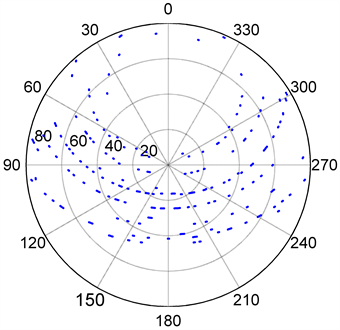Figure 3. Distribution of the measured pointing error in horizontal coordinates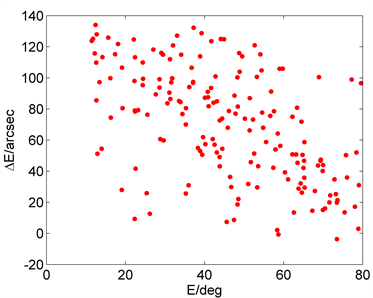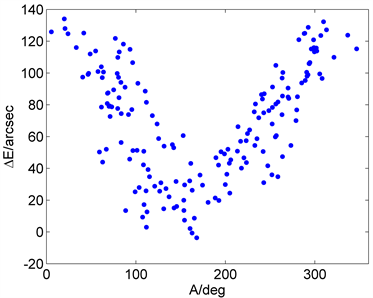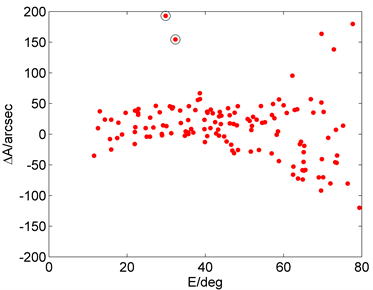Figure 4. The varies of extracted azimuth error ΔA and elevation error ΔE with azimuth A and elevation E

$\left\{\begin{array}{l}{\delta }_{A}=\sqrt{\sum {\left(\Delta A\mathrm{cos}E\right)}^{2}/{n}_{A}}\\ {\delta }_{E}=\sqrt{\sum {\left(\Delta E\right)}^{2}/{n}_{E}}\end{array}$ (17)

$\delta =\sqrt{{\left({\delta }_{A}\right)}^{2}+{\left({\delta }_{E}\right)}^{2}}$ (18)

“提取算法1”得得到的天线方位指向误差均方根δA = 28.69″，俯仰指向误差均方根δE = 78.45″，指向总误差δ = 83.53″。“提取算法2”得到的天线方位指向误差均方根δA = 21.64″，俯仰指向误差均方根δE = 78.22″，指向总误差δ = 81.16″。其中“提取算法1”与“提取算法2”主要差别在于方位指向误差均方根相差较大，这主要是由于“提取算法1”引入了两个明显的离群点所致(如图4“o”所标注)。此外可知若不对射电望远镜的指向进行误差修正，则其指向精度不能满足实际观测要求。下面将得到的初始误差数据分别利用式(4)的指向模型进行模型误差修正。

4.2. 模型误差修正结果分析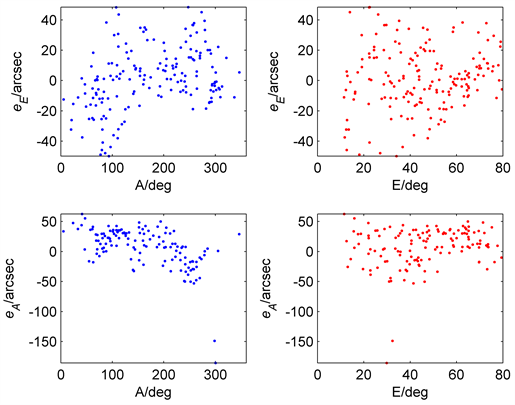(a) “提取算法1”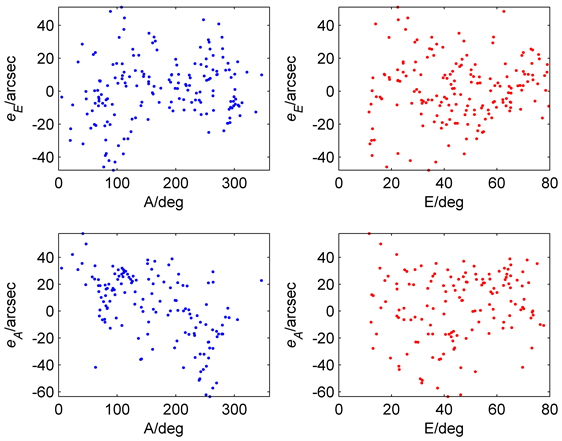(b) “提取算法2”

Figure 5. The varies of calibrated azimuth error eA and elevation error eE with azimuth A and elevation E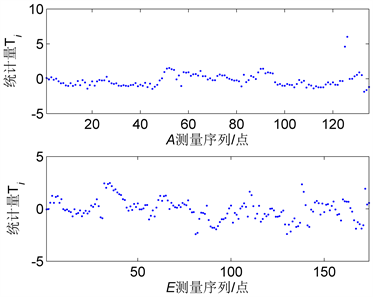(a) “提取算法1”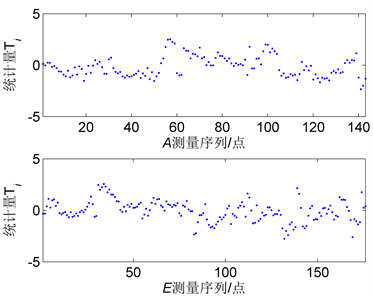(b) “提取算法2”

Figure 6. The varies of Statistics Ti from the measured error with azimuth A and elevation E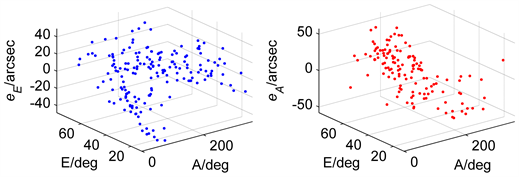(a) “提取算法1”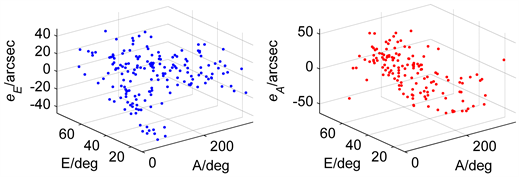(b) “提取算法2”

Figure 7. The varies of calibrated azimuth error eA and elevation error eE with azimuth A and elevation E

4.3. 模型参数显著性检验结果分析Table 1. The hypothesis test of the adopted model

5. 结论

  董光亮, 雷厉. 中国深空网: 系统设计与关键技术(上)S/X双频段深空测控通信系统[M]. 北京: 清华大学出版社, 2016: 89-129.  高冠男. 云台40米射电望远镜天线控制系统运行测试分析及天线指向误差校正[D]: [硕士学位论文]. 云南: 中国科学院研究生院(云南天文台), 2007.  刘璇, 艾力•玉苏甫, 项斌斌, 等. 轨道不平度对天线指向的影响分析与修正[J]. 天文学报, 2017, 58(4): 49-59.  雒小红. 天线角编码误差高精度修正方法[J]. 通讯世界, 2020, 27(2): 110-111.  付丽, 凌权宝, 赵融冰, 等. TM65m天线基础和轨道沉降及对天线指向的影响[J]. 红外与激光工程, 2016, 45(11): 190-196.  温浩兴, 许谦, 王娜. 南山26米射电望远镜轨道高差测量及其对指向精度的影响[J]. 天文研究与技术, 2019, 16(2): 158-166.  Hu, K.Y., Yusup, A.L. and XU, Q. (2015) Deformation Measurement and Pointing Model Analysis of 25 m Antenna. Applied Mechanics and Materials, 713-715, 424-431. https://doi.org/10.4028/www.scientific.net/AMM.713-715.424  Kong, D.Q., Wang, S.G., Wang, J.Q., et al. (2014) A New Calibration Model for Pointing a Radio Telescope that Considers Nonlinear Errors in the Azimuth Axis. Research in Astronomy and Astrophysics, 14, 733-740. https://doi.org/10.1088/1674-4527/14/6/011  Zhang, J., Huang, J., Liang, W., Wang, C., et al. (2018) A Correction Method of Estimating the Pointing Error for Reflector Antenna. Shock and Vibration, 2018, Article ID: 3262869. https://doi.org/10.1155/2018/3262869  Meeks, M., Ball, J. and Hull, A. (1968) The Pointing Calibration of the Haystack Antenna. IEEE Transactions on Antennas & Propagation, 16, 746-751. https://doi.org/10.1109/TAP.1968.1139287  武宇翔, 张洪波, 孔德庆, 等. 基于实时参数的大气折射模型和射电望远镜指向修正方法[J]. 光子学报, 2019, 48(8): 42-49.  姚克荣, 许洪韬, YAO, 等. 大型高精度天线座轨道的安装方法研究[J]. 电子机械工程, 2018, 34(191): 58-61+68.  施浒立, 古天龙. 雷达天线和天文望远镜指向误差修正的数学模型——拟里兹广义插值[J]. 电子机械工程, 1987(5):1-7.  高冠男, 汪敏, 施硕彪, 等. 云台40 m射电望远镜的指向误差校正[J]. 天文研究与技术, 2007, 4(2): 188-194.  赵军祥, 教富龙, 杨文洁. 利用射电星校准13m测控天线指向误差及测试结果[J]. 飞行器测控学报, 2009, 28(3): 13-15.  姜正阳, 孔德庆, 张洪波, 等. 考虑轨道不平度的射电望远镜指向修正方法[J]. 天文研究与技术, 2015, 12(4): 417-423.  何晓群, 刘文卿. 应用回归分析[M]. 北京: 科学出版社, 2020: 66-119.